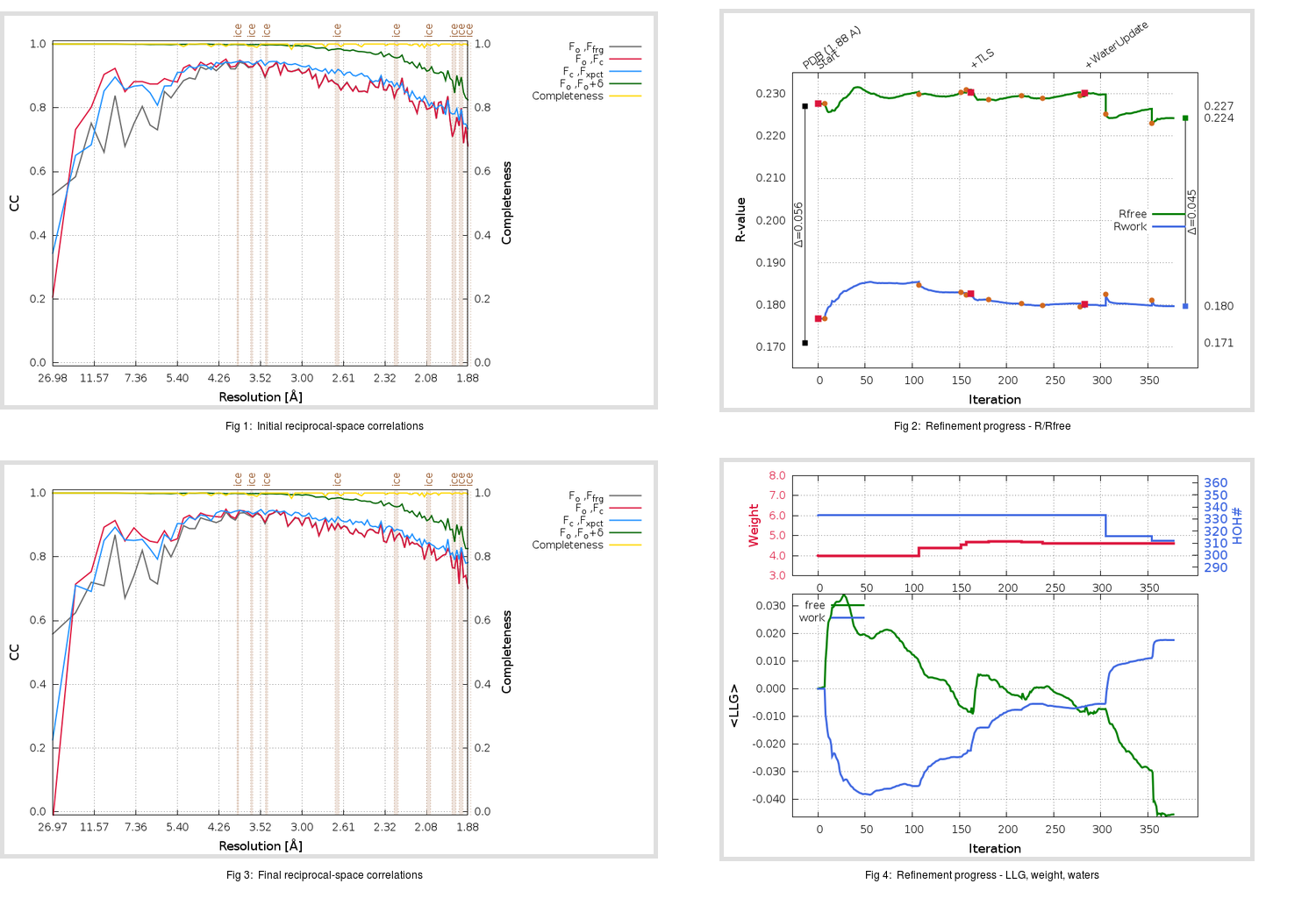Content:

```    Diffraction limits & principal axes of ellipsoid fitted to diffraction cut-off surface:
1.856         0.9267   0.0000   0.3757       0.998 a* + 0.067 c*
1.821         0.0000   1.0000   0.0000       b*
1.802        -0.3757   0.0000   0.9267      -0.694 a* + 0.720 c*
```

## Deposited

` `
 Date deposited Date data collection Resolution R, Rfree 20200315 20200304 1.88 0.1680 0.2270

Molprobity (CCP4 7.0 version) summary:

```Ramachandran outliers =   0.33 %
favored =  97.35 %
Rotamer outliers      =   1.14 %
C-beta deviations     =     0
Clashscore            =   6.34
RMS(bonds)            =   0.0130
RMS(angles)           =   1.86
MolProbity score      =   1.52
Resolution            =   1.88
R-work                =   0.1680
R-free                =   0.2270
```

```Number of waters      =   333

<B> (all atoms) =   26.06 ( sd =    9.42 ) for       2729 non-hydrogen atoms
<B>   (protein) =   24.56 ( sd =    8.49 ) for       2370 non-hydrogen atoms
<B>     (water) =   35.97 ( sd =    9.56 ) for        333 non-hydrogen atoms
<B>    (others) =   36.77 ( sd =   10.49 ) for         26 non-hydrogen atoms

B min/max       (all non-hydrogen atoms) =   14.01 /   71.98
B min/max   (protein non-hydrogen atoms) =   14.01 /   71.98
B min/max     (water non-hydrogen atoms) =   14.53 /   62.89
B min/max     (other non-hydrogen atoms) =   28.91 /   45.66
```

## BUSTER (re-)refinement

` `

Molprobity (CCP4 7.0 version) summary:

```Ramachandran outliers =   0.33 %
favored =  98.34 %
Rotamer outliers      =   1.89 %
C-beta deviations     =     0
Clashscore            =   2.96
RMS(bonds)            =   0.0114
RMS(angles)           =   1.56
MolProbity score      =   1.30
Resolution            =   1.88
R-work                =   0.1797
R-free                =   0.2242
```

```Number of waters      =   312

<B> (all atoms) =   26.10 ( sd =    8.91 ) for       2708 non-hydrogen atoms
<B>   (protein) =   24.42 ( sd =    7.25 ) for       2370 non-hydrogen atoms
<B>     (water) =   38.13 ( sd =   10.52 ) for        312 non-hydrogen atoms
<B>    (others) =   35.98 ( sd =   12.18 ) for         26 non-hydrogen atoms

B min/max       (all non-hydrogen atoms) =   12.87 /   76.60
B min/max   (protein non-hydrogen atoms) =   12.87 /   63.49
B min/max     (water non-hydrogen atoms) =   14.33 /   76.60
B min/max     (other non-hydrogen atoms) =   28.35 /   47.84
```

Refinement progression:Results:

` `
 File Remark 5REO_aB_refine.01_03_refine.pdb.gz exact refinement commands are in header 5REO_aB_refine.01_03_refine.mtz.gz including original deposited data and several re-refinement map coefficients 5REO_aB_refine.01_03_BUSTER_model.cif.gz including any non-standard compound restraints 5REO_aB_refine.01_03_BUSTER_refln.cif.gz